Learning Library

# Math Facts: Fishing For Answers

Go fishing for math fact families with this colorful series of 1st grade worksheets.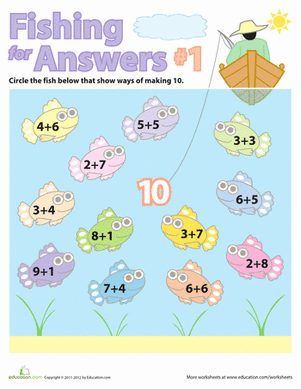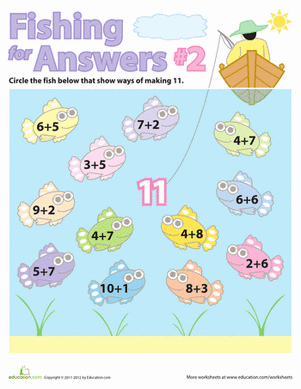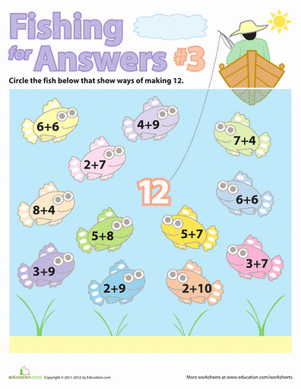There are plenty of fish in the sea! But only a few add together to make 12! This fun worksheet will help your child practice his simple addition.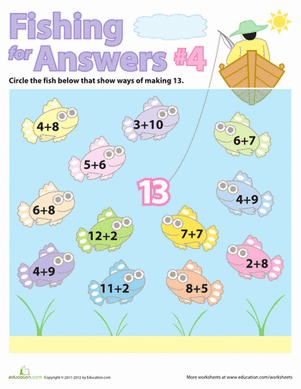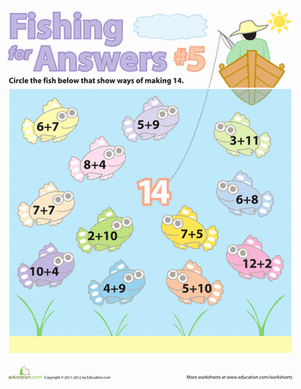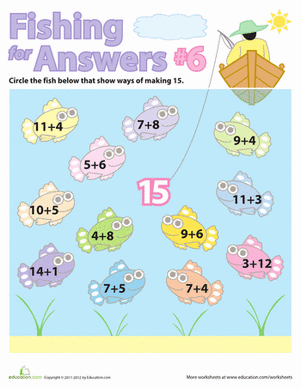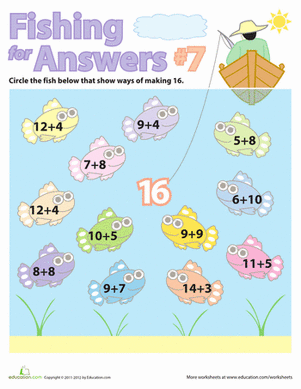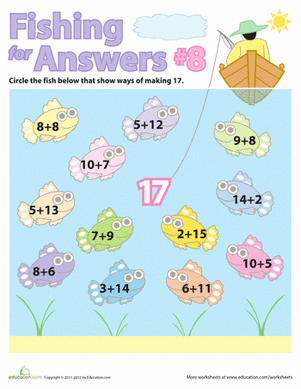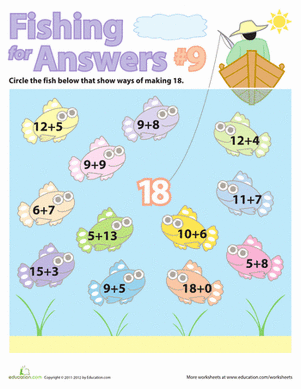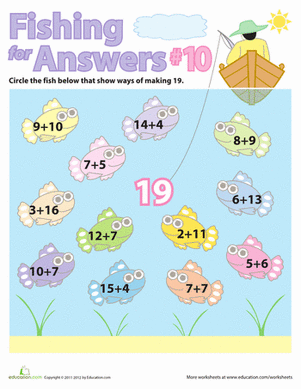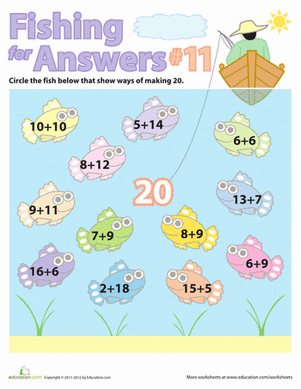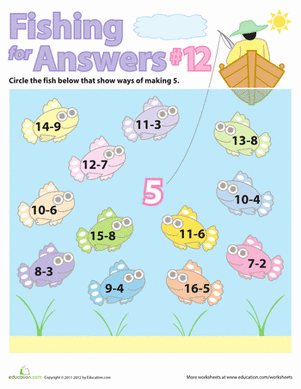Your child will get to "Fish for Answers" with this fun math facts worksheet!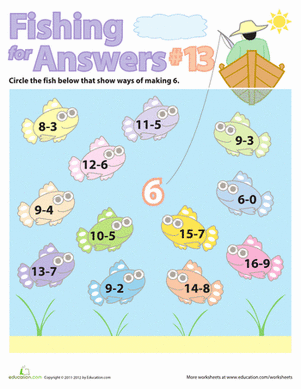Your child will get to "Fish for Answers" with this fun subtraction worksheet, a great way to practice subtraction math facts!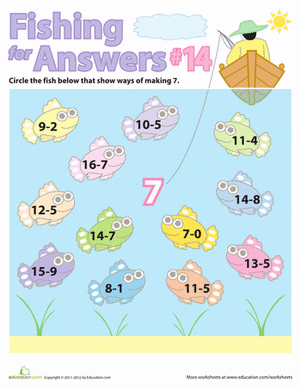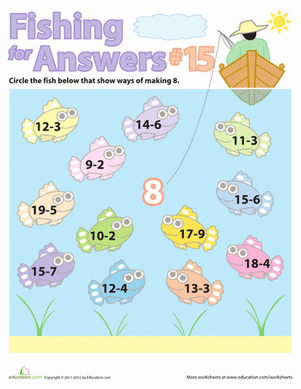Play the "Fish for Answers" game, and make subtraction seem easy! Find all the equations that equal eight.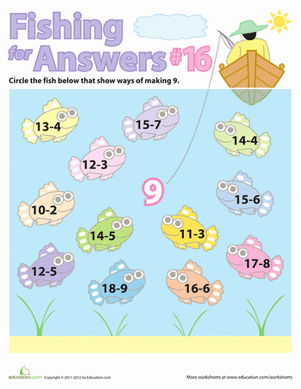Go fishing with a fun math worksheet! Your first-grader can practice visualizing all the ways to subtract one and two digit numbers to equal nine.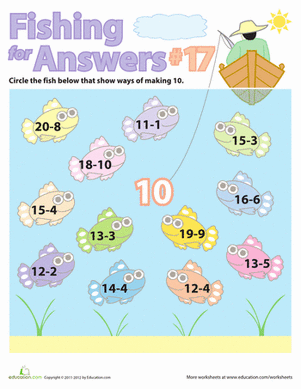Practice simple subtraction with your child. She can play the "Fish for Answers" game to learn math facts!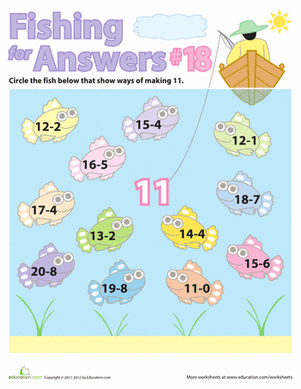If math is becoming a bummer, just go fishing! This fun game will be perfect for introducing your first grader to simple subtraction equations.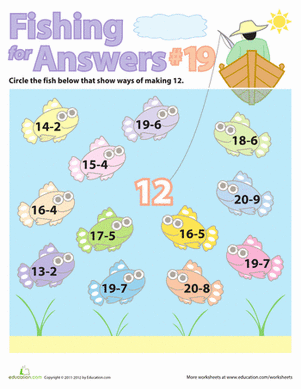Introduce your child to simple subtraction with a fun "Fish for Answers" math game!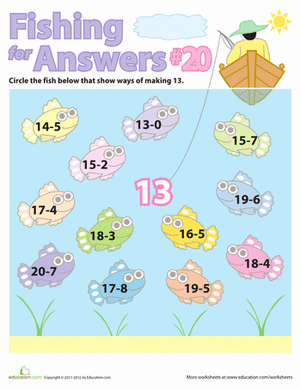Practice solving simple subtraction problems with a fun game! You and your child can "fish for answers" to find all the ways to make the number thirteen.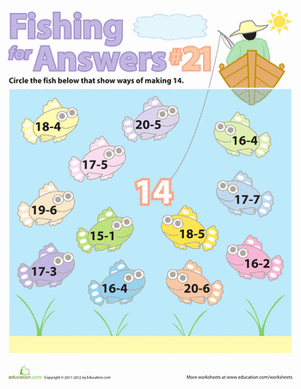You and your first grader will get to "Fish for Answers" with this fun subtraction facts worksheet.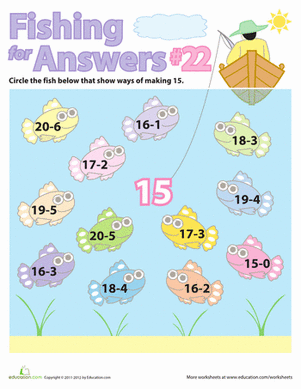Give your child a break from boring math facts, and challenge her with a fun subtraction game!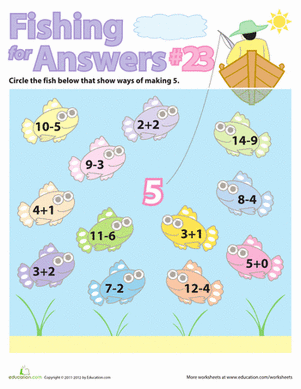Make math fun with this series of "Fishing for Answers" math facts!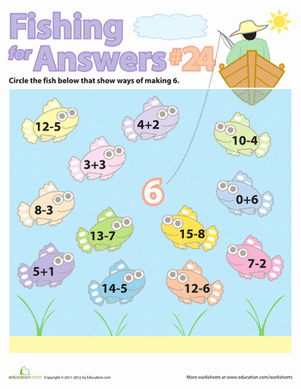Give your child a great game for practicing math facts! She'll "fish" for the right answer by solving all the simple subtraction and addition equations.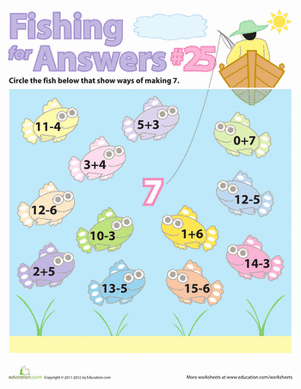Go fishing for math facts with a fun game! Your first grader will practice solving simple addition and subtraction problems.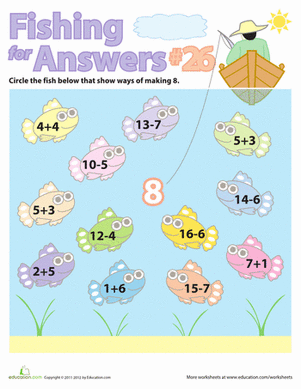Make math fun with this series of "Fishing for Answers" addition and subtraction math facts.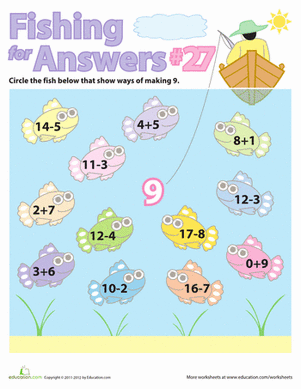Give your first grader a great visual learning game, where he can "Fish for Answers" and learn his math facts!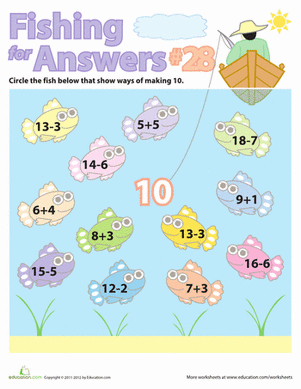Math got your child down in the dumps? Just go fishing! Play the "Fish for Answers" game to learn simple addition and subtraction.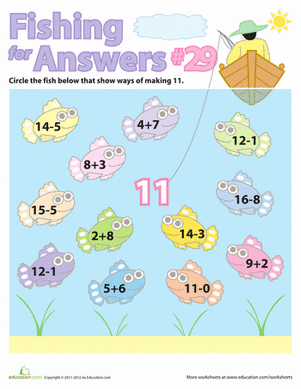Make math facts fun with this series of "Fishing for Answers" addition and subtraction games!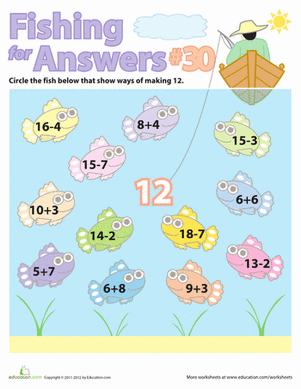How many different ways can you add and subtract to make the number twelve? Find out with a fun "Fishing for Answers" math facts game!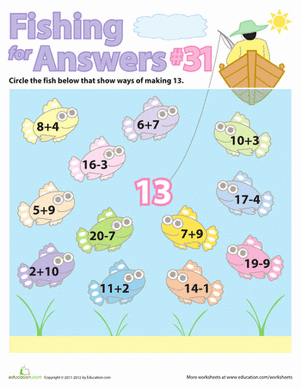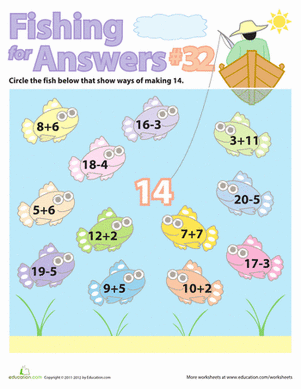Make math fun with this series of "Fishing for Answers" math facts. Your child will be able to practice doing simple subtraction and addition equations.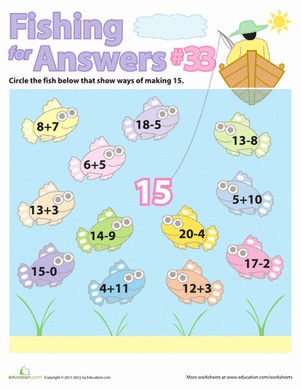Does your child need a jump-start on simple arithmetic? Give her a great game to get started learning addition and subtraction math facts!

Create new collection

0

### New Collection>

0 items

What could we do to improve Education.com?